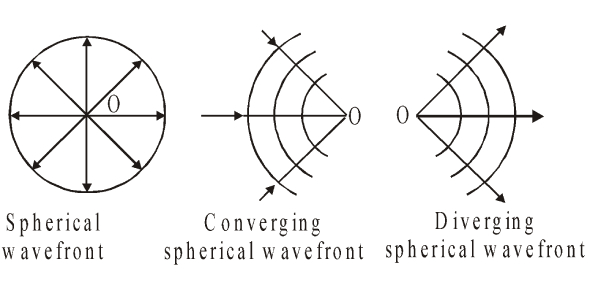# How Much You Know About Wave Optics? Trivia Quiz

19 Questions | Attempts: 1407SettingsWave optics is a division of optics that studies interference, diffraction, polarization, and other phenomena for which the approximation of geometric optics is not valid. This usage tends not to include quantum noise in optical communication, studied in the sub-branch of coherence theory. Confusing? This quiz might clear up your questions.

• 1.
Only the wave theory of light offers an explanation for the ability of light to exhibit
• A.

Interference

• B.

Reflection

• C.

Photoelectric emission

• D.

• 2.
Which two characteristics of light can best be explained by the wave theory of light?
• A.

Relection and refraction

• B.

Reflection and interference

• C.

Refraction and diffraction

• D.

Interference and diffraction

• 3.
Which phenomenon can be observed for transverse waves only?
• A.

Reflection

• B.

Diffraction

• C.

Polarization

• D.

Refraction

• 4.
Sources that produce waves with a constant phase relation are said to be
• A.

Polarized

• B.

Diffused

• C.

Refracted

• D.

Coherent

• 5.
If all parts of a light beam have a constant phase relationship, with the same wavelength and frequency, the light beam is
• A.

Monochromatic and coherent

• B.

Mono and incoherent

• C.

Polychromatic and coherent

• D.

Poly and incoherent

• 6.
The diffraction pattern produced by a double-slit will show greatest separation of maxima when the color of the light source is
• A.

Red

• B.

Orange

• C.

Blue

• D.

Green

• 7.
If the wavelength of the light is decreased, the width of the central maximum in the diffraction pattern will
• A.

Decrease

• B.

Increase

• C.

Remain the same

• 8.
A wave is diffracted as it passes through an opening in a barrier. The amount of diffraction that the wave undergoes depends on both the
• A.

Amplitude and frequency of the incident wave

• B.

Wavelength and speed of the incident wave

• C.

Wavelength of the incident wave and size of the opening

• D.

Amplitude of the incicdent wave and the size of the opening

• 9.
If the screen is moved closer to the slits, the distance between the central maximum and the first maximum will
• A.

Decrease

• B.

Increase

• C.

Remain the same

• 10.
If the distance between the slits is decreased, the distance between the central max and the first max will
• A.

Decrease

• B.

Increase

• C.

Remain the same

• 11.
The photon model of light is more appropriate than the wave model in explaining.
• A.

Interference

• B.

Refraction

• C.

Polarization

• D.

Photoelectric emission

• 12.
Light demonstrates the characteristics of
• A.

Particles only

• B.

Waves only

• C.

Both particles and waves

• D.

Neither

• 13.
Interference and diffraction can be explained by
• A.

The wave theory only

• B.

The particle theory only

• C.

Neither

• D.

Both

• 14.
The color of visible light is determined by its
• A.

Frequency

• B.

Amplitude

• C.

Intensity

• D.

Speed

• 15.
Increasing the amplitude of an electromagnetic radiation increases its
• A.

Frequency

• B.

Speed

• C.

Intensity

• D.

Period

• 16.
Compared to the period of red light, the period of violet light is
• A.

Less

• B.

Greater

• C.

Same

• 17.
Compared to the period of a wave of red light, the period of a wave of blue light is
• A.

Less

• B.

Greater

• C.

Same

• 18.
Electrons oscillating with a frequency of 2.0X10 10 hertz produce electromagnetic waves. These waves would be classified as
• A.

Infrared

• B.

Visible

• C.

Microwave

• D.

X-ray

• 19.
Which pair of terms best describes light waves traveling from the Sun to Earth?
• A.

Electromagnetic and transverse

• B.

Electromagnetic and longitudinal

• C.

Mechanical and transverse

• D.

Mechanical and longitudinal

## Related TopicsBack to top
×

Wait!
Here's an interesting quiz for you.